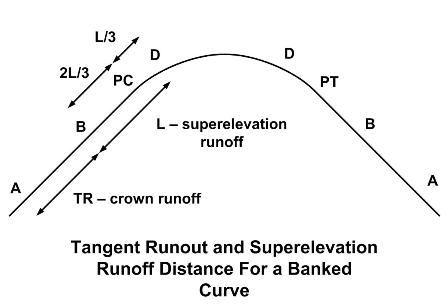* Log In to use the Calculate function * Become a Member!

Calculate the Tangent Runout And Superelevation Runoff Distance For A Banked Curve:

Calculation:
Designer/Checker:

Input:Road lane width (w - ft or m)
Road cross slope (p - ft/ft or m/m)
Superelevation runoff rate (SRR)*
Curve superelevation (e - ft/ft or m/m)
*An SRR of 1/200 = .005 is commonly used for for speeds less than 50 mph.

Output: